# RS Aggarwal Solutions for Class 7 Maths Exercise 17B Chapter 17 Constructions

RS Aggarwal Solutions for Class 7 Maths Exercise 17B Chapter 17 Constructions, in this exercise all questions are included, which will help students to have a better understanding of the concepts and let them develop problem-solving abilities. This RS Aggarwal Solutions for Class 7 Maths Chapter 17, can also be used by the students who appear for any competitive exams. The topics covered in this exercise are the construction of triangles, such as SSS triangle construction, SAS triangle construction, ASA triangle construction and RHS triangle construction. The RS Aggarwal Solutions for Class 7 Maths Chapter 17 Constructions, are designed by our experts in BYJU’S, based on CBSE syllabus.

## Download the PDF of RS Aggarwal Solutions For Class 7 Maths Chapter 17 Constructions – Exercise 17B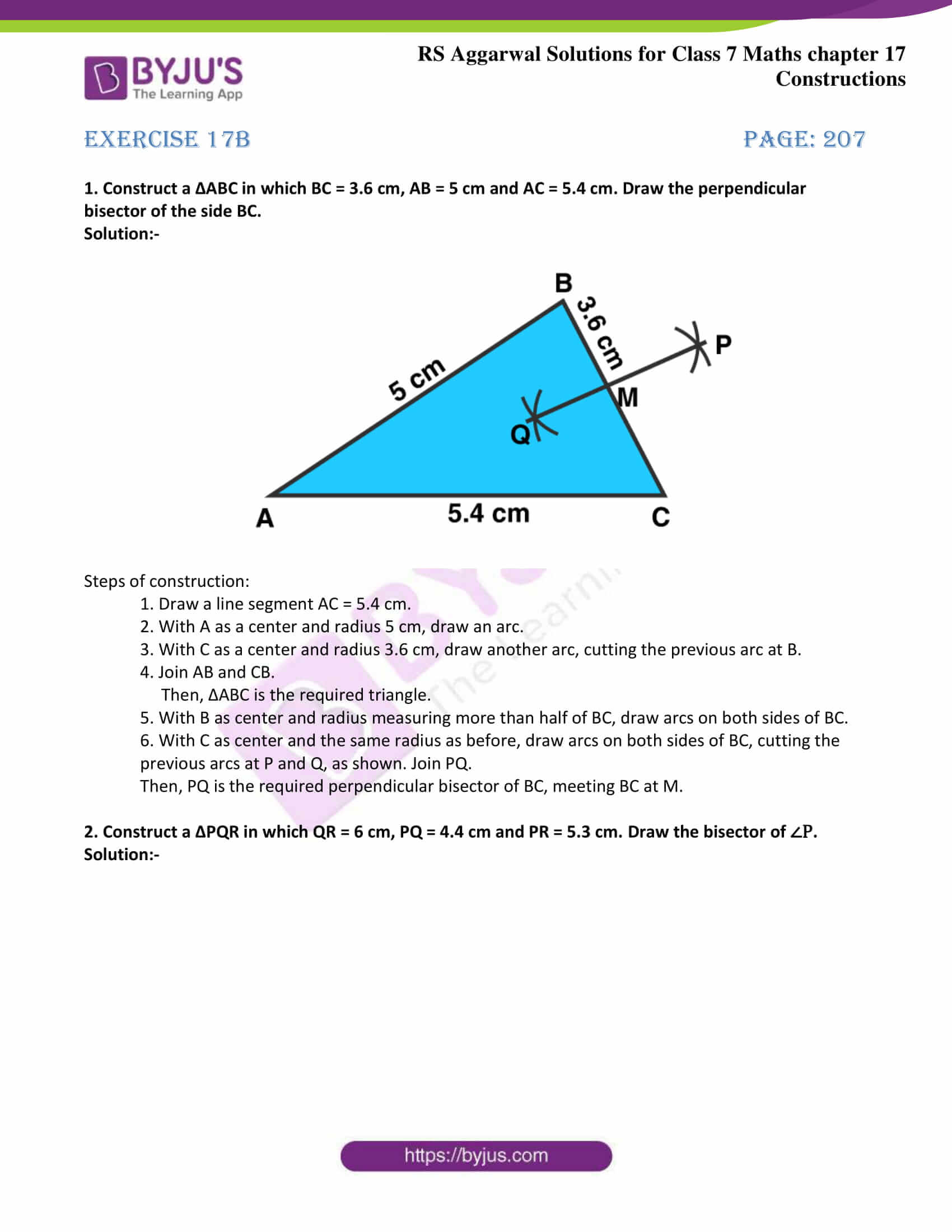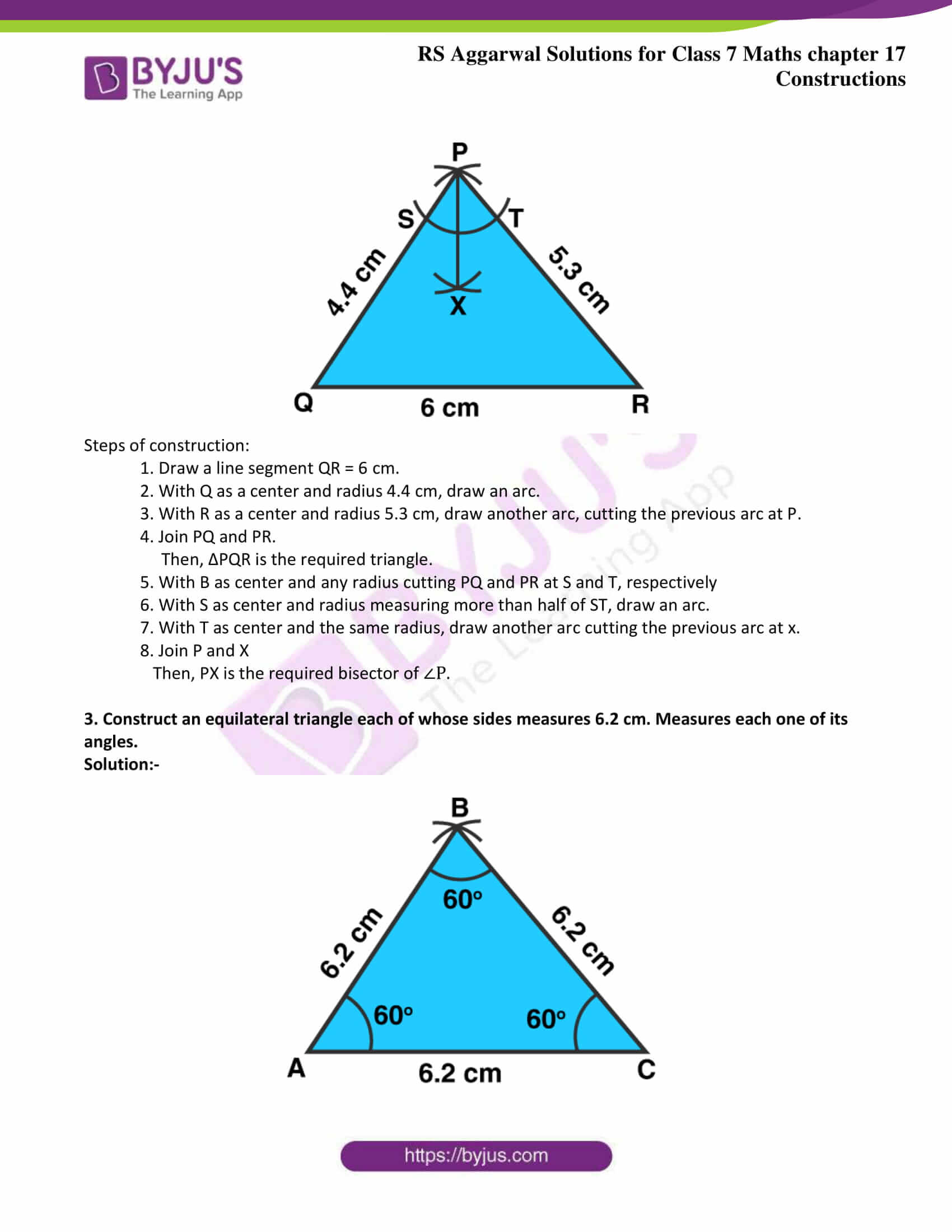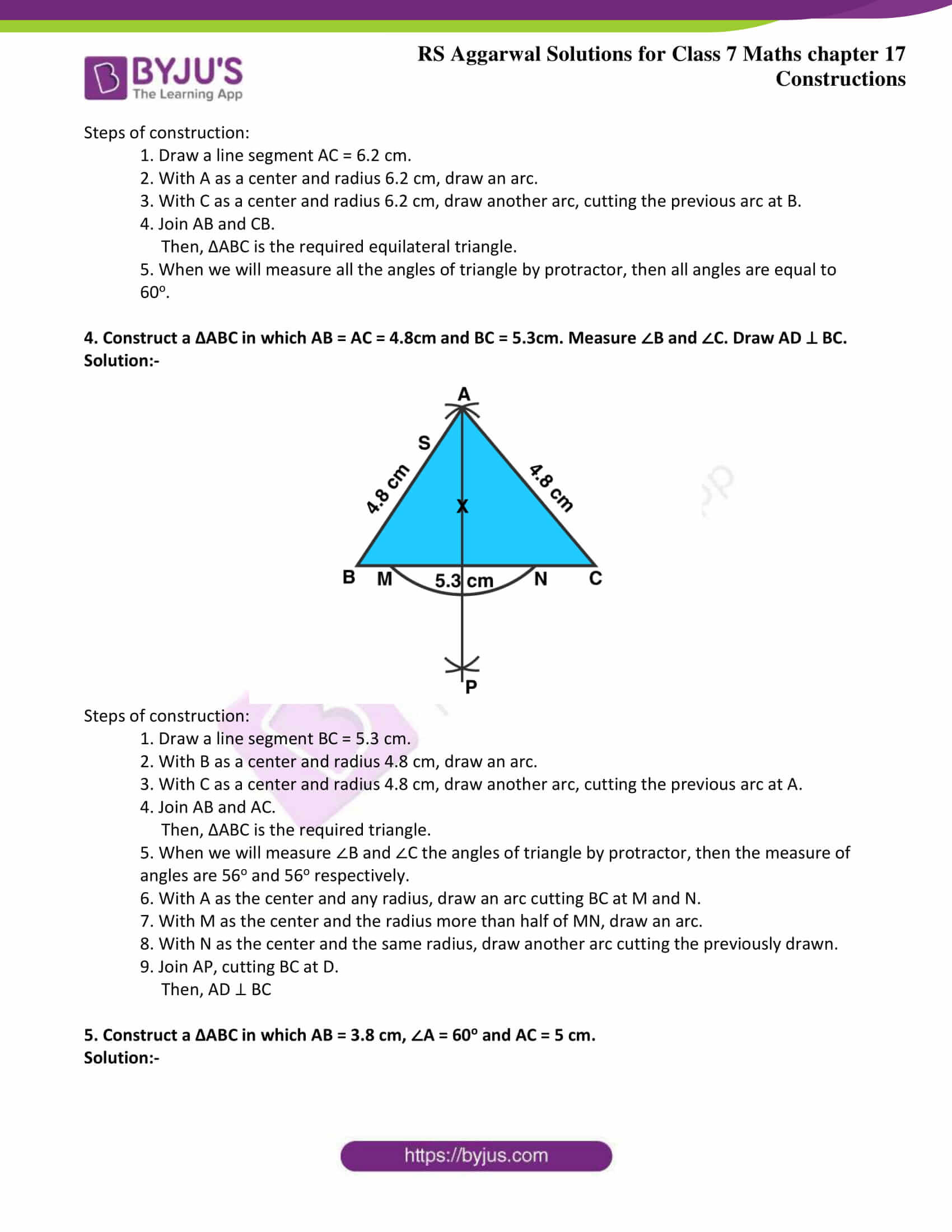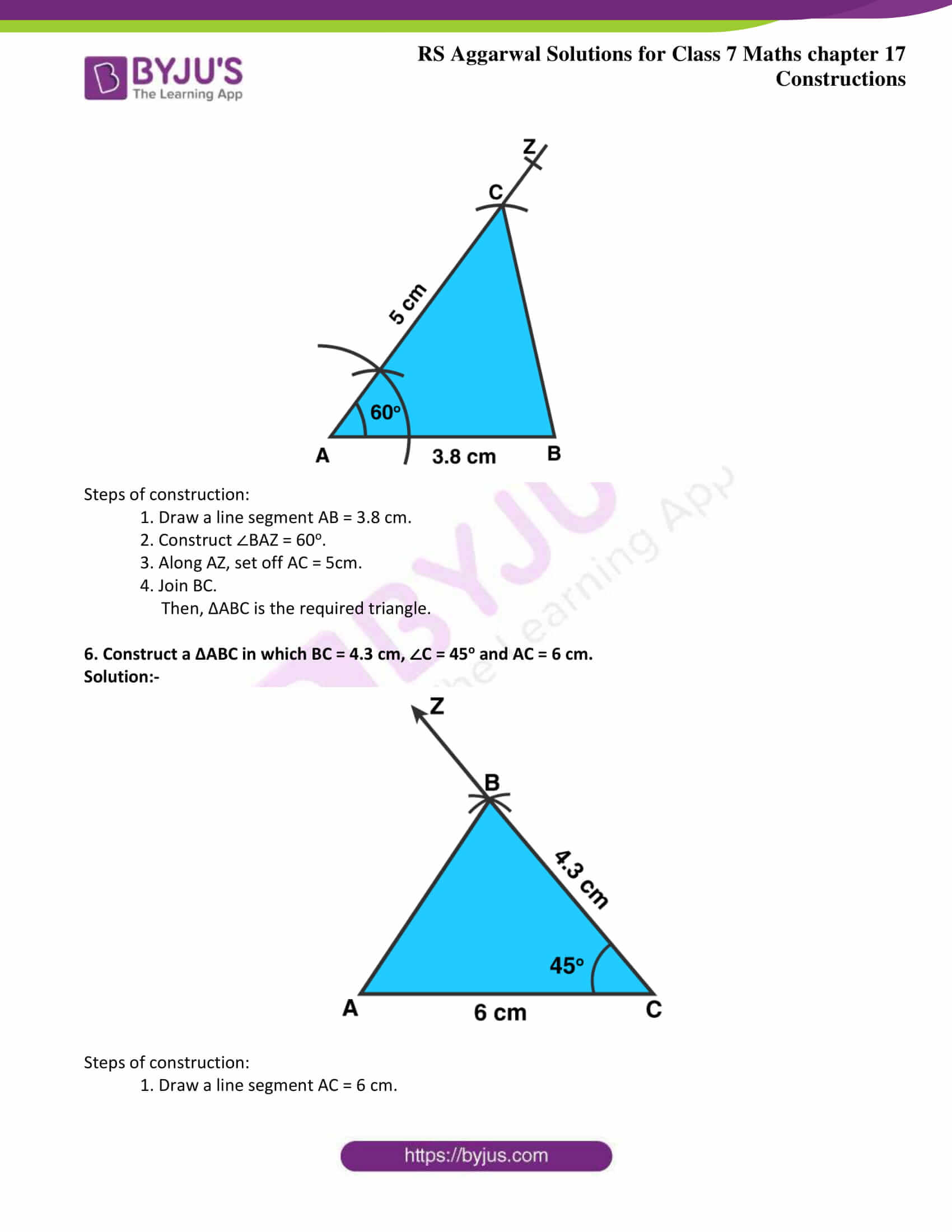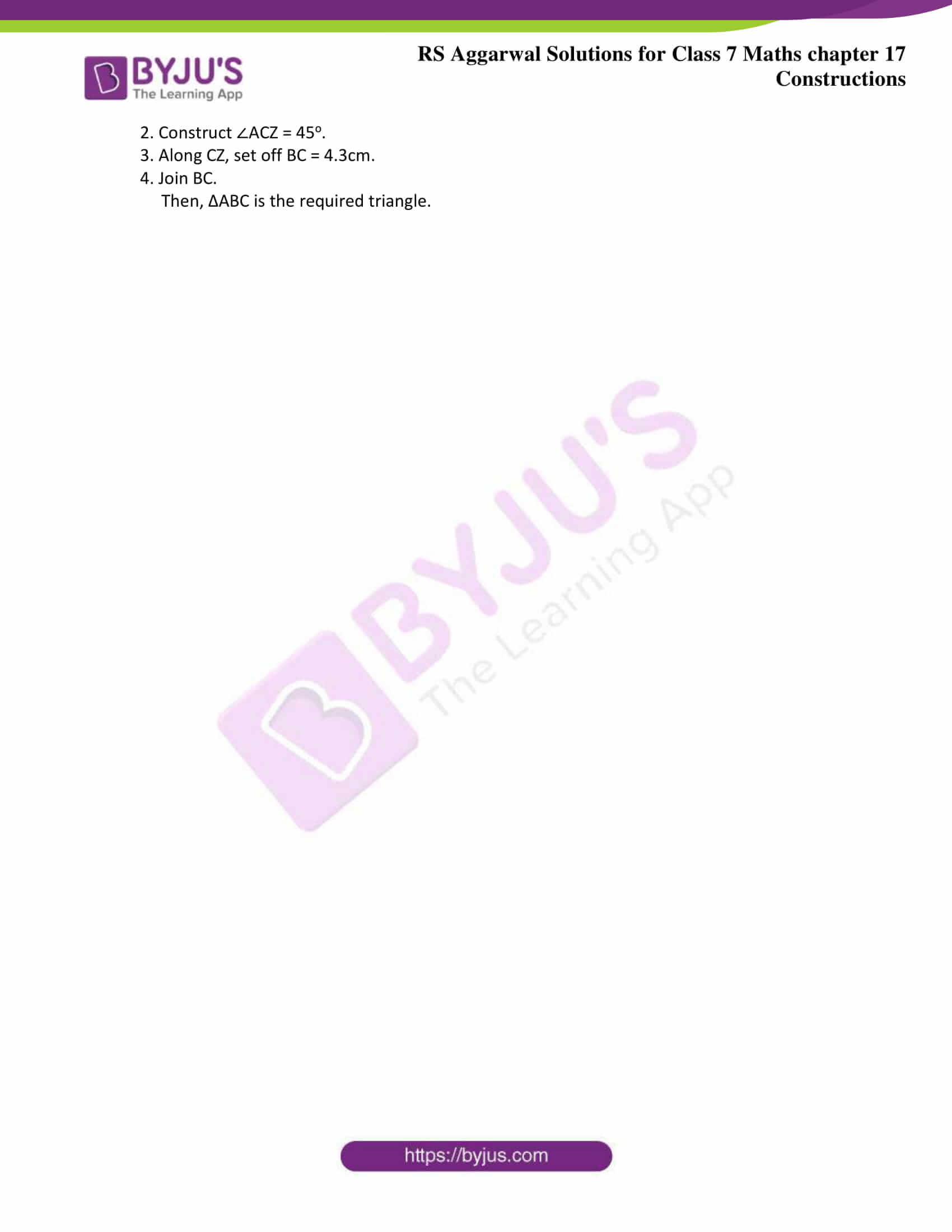### Access answers to Maths RS Aggarwal Solutions for Class 7 Chapter 17 – Constructions Exercise 17B

1. Construct a ΔABC in which BC = 3.6 cm, AB = 5 cm and AC = 5.4 cm. Draw the perpendicular bisector of the side BC.

Solution:-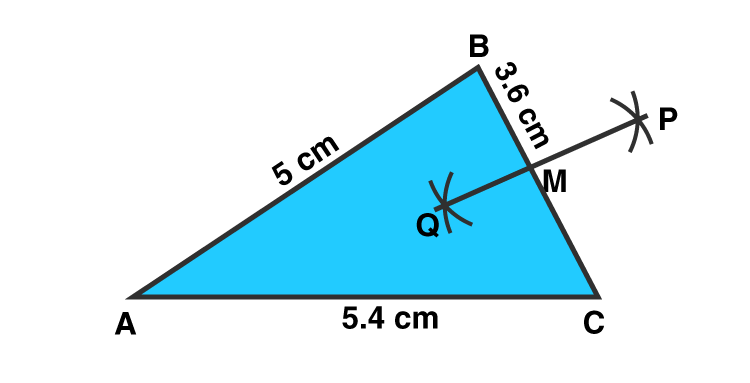Steps of construction:

1. Draw a line segment AC = 5.4 cm.

2. With A as a center and radius 5 cm, draw an arc.

3. With C as a center and radius 3.6 cm, draw another arc, cutting the previous arc at B.

4. Join AB and CB.

Then, ΔABC is the required triangle.

5. With B as center and radius measuring more than half of BC, draw arcs on both sides of BC.

6. With C as center and the same radius as before, draw arcs on both sides of BC, cutting the previous arcs at P and Q, as shown. Join PQ.

Then, PQ is the required perpendicular bisector of BC, meeting BC at M.

2. Construct a ΔPQR in which QR = 6 cm, PQ = 4.4 cm and PR = 5.3 cm. Draw the bisector of ∠P.

Solution:-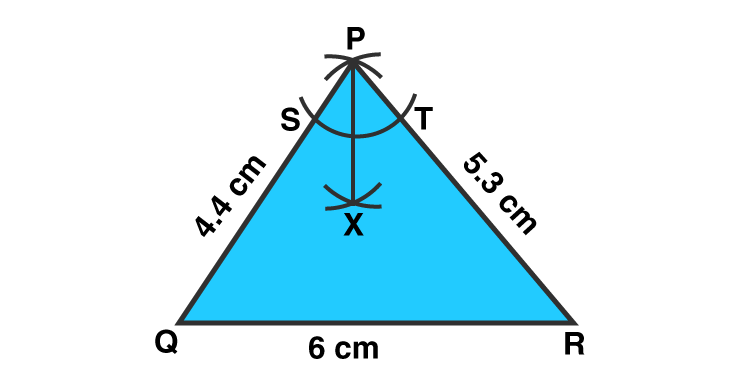Steps of construction:

1. Draw a line segment QR = 6 cm.

2. With Q as a center and radius 4.4 cm, draw an arc.

3. With R as a center and radius 5.3 cm, draw another arc, cutting the previous arc at P.

4. Join PQ and PR.

Then, ΔPQR is the required triangle.

5. With B as center and any radius cutting PQ and PR at S and T, respectively

6. With S as center and radius measuring more than half of ST, draw an arc.

7. With T as center and the same radius, draw another arc cutting the previous arc at x.

8. Join P and X

Then, PX is the required bisector of ∠P.

3. Construct an equilateral triangle each of whose sides measures 6.2 cm. Measures each one of its angles.

Solution:-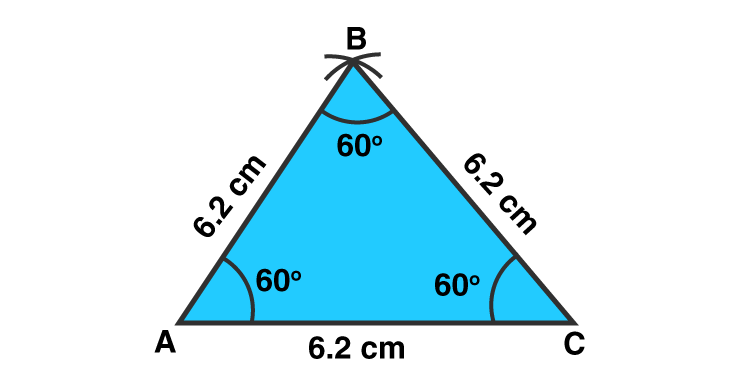Steps of construction:

1. Draw a line segment AC = 6.2 cm.

2. With A as a center and radius 6.2 cm, draw an arc.

3. With C as a center and radius 6.2 cm, draw another arc, cutting the previous arc at B.

4. Join AB and CB.

Then, ΔABC is the required equilateral triangle.

5. When we will measure all the angles of triangle by protractor, then all angles are equal to 60o.

4. Construct a ΔABC in which AB = AC = 4.8cm and BC = 5.3cm. Measure ∠B and ∠C. Draw AD ⊥ BC.

Solution:-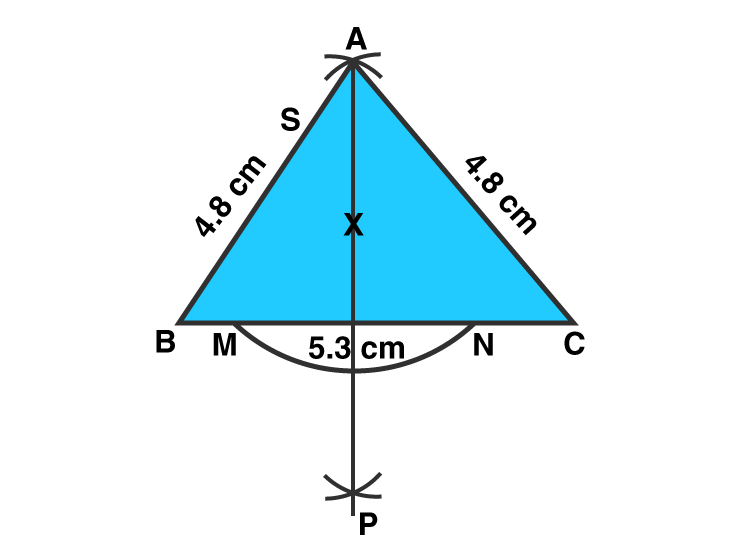Steps of construction:

1. Draw a line segment BC = 5.3 cm.

2. With B as a center and radius 4.8 cm, draw an arc.

3. With C as a center and radius 4.8 cm, draw another arc, cutting the previous arc at A.

4. Join AB and AC.

Then, ΔABC is the required triangle.

5. When we will measure ∠B and ∠C the angles of triangle by protractor, then the measure of angles are 56o and 56o respectively.

6. With A as the center and any radius, draw an arc cutting BC at M and N.

7. With M as the center and the radius more than half of MN, draw an arc.

8. With N as the center and the same radius, draw another arc cutting the previously drawn.

9. Join AP, cutting BC at D.

5. Construct a ΔABC in which AB = 3.8 cm, ∠A = 60o and AC = 5 cm.

Solution:-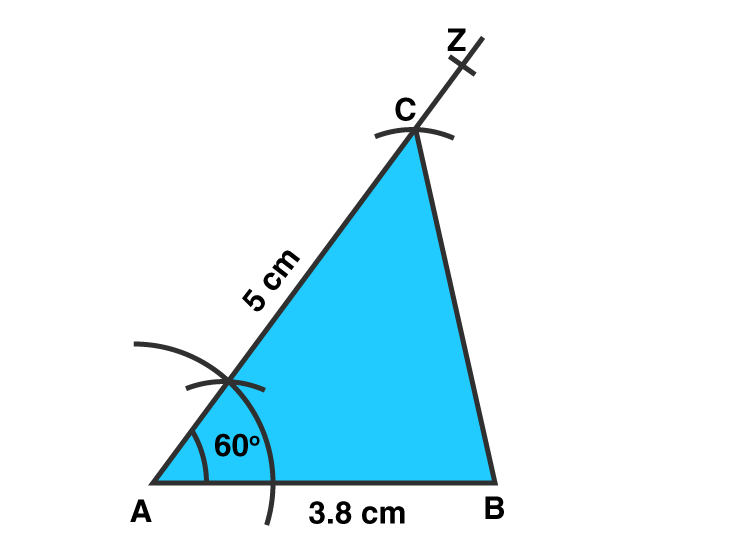Steps of construction:

1. Draw a line segment AB = 3.8 cm.

2. Construct ∠BAZ = 60o.

3. Along AZ, set off AC = 5cm.

4. Join BC.

Then, ΔABC is the required triangle.

6. Construct a ΔABC in which BC = 4.3 cm, ∠C = 45o and AC = 6 cm.

Solution:-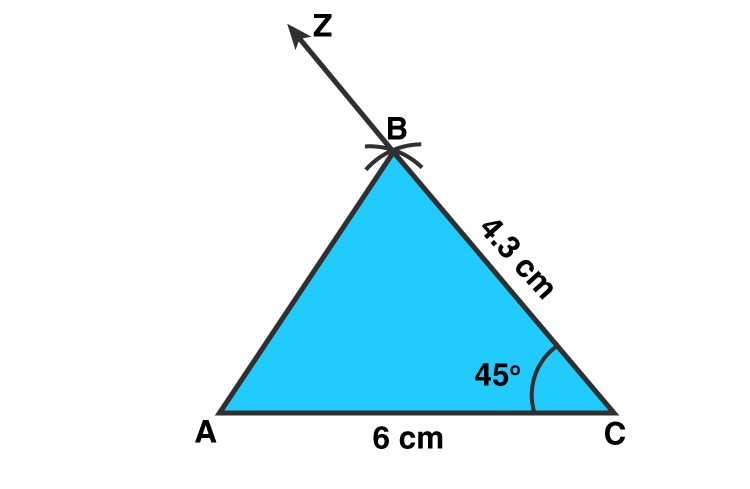Steps of construction:

1. Draw a line segment AC = 6 cm.

2. Construct ∠ACZ = 45o.

3. Along CZ, set off BC = 4.3cm.

4. Join BC.

Then, ΔABC is the required triangle.

### Access other exercises of RS Aggarwal Solutions For Class 7 Chapter 17 – Constructions

Exercise 17A Solutions

Exercise 17C Solutions